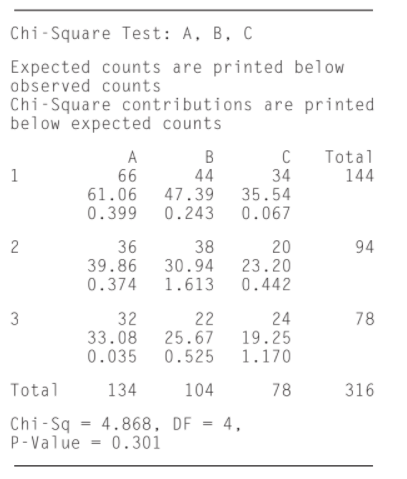×
Get Full Access to Statistics For Engineers And Scientists - 4 Edition - Chapter 6.10 - Problem 14e
Get Full Access to Statistics For Engineers And Scientists - 4 Edition - Chapter 6.10 - Problem 14e

×

# At a certain genetic locus on a chromosome, eachISBN: 9780073401331 38

## Solution for problem 14E Chapter 6.10

Statistics for Engineers and Scientists | 4th Edition

• Textbook Solutions
• 2901 Step-by-step solutions solved by professors and subject experts
• Get 24/7 help from StudySoup virtual teaching assistantsStatistics for Engineers and Scientists | 4th Edition

4 5 1 323 Reviews
22
4
Problem 14E

At a certain genetic locus on a chromosome, each individual has one of three different DNA sequences (alleles). The three alleles are denoted A, B, C. At another genetic locus on the same chromosome, each organism has one of three alleles, denoted 1, 2, 3. Each individual therefore has one of nine possible allele pairs: A1, A2, A3, B1, B2, B3, C1, C2, or C3. These allele pairs are called haplotypes. The loci are said to be in linkage equilibrium if the two alleles in an individual’s haplotype are independent. Haplotypes were determined for 316 individuals. The following MINITAB output presents the results of a chi-square test for independence.a. How many individuals were observed to have the haplotype B3?

b. What is the expected number of individuals with the haplotype A2?

c. Which of the nine haplotypes was least frequently observed?

d. Which of the nine haplotypes has the smallest expected count?

e. Can you conclude that the loci are not in linkage equilibrium (i.e., not independent)? Explain.

f. Can you conclude that the loci are in linkage equilibrium (i.e., independent)? Explain.

Step-by-Step Solution:
Step 1 of 3

Genetics: Week 2 Chapter 2: Mendelian Inheritance Cont. I. Genetics and Probability Number of times an event occurs a. Probability = Total number of events b. Eugenics: Trying to make sure positive genes stay in the gene pool i. Advising couples in a way to ensure this c. Euthenics: Give the right environment for a population to thrive d. Euphenics: Improving the phenotype (beautifying the phenotype) e. The accuracy of the probability prediction depends largely on the size of the sample f. Often there is a deviation between observed and expected values (random sampling error) i. The smaller the sampling size, the larger the error and vice versa g. To compute probability, 3 mathematical operations can be used i. Sum rule 1. “Either or” 2. Probability that one of two or more mutually exclusive events will occur is the sum of their individual probabilities ii. Product rule 1. “And:” more than one outcome 2. Will often have an order but not always 3. Multiply the probabilities together iii. Binomial Expansion equation 1. Used in problems involving unordered events 2. h. Chi Square: Test the

Step 2 of 3

Step 3 of 3

##### ISBN: 9780073401331

The answer to “?At a certain genetic locus on a chromosome, each individual has one of three different DNA sequences (alleles). The three alleles are denoted A, B, C. At another genetic locus on the same chromosome, each organism has one of three alleles, denoted 1, 2, 3. Each individual therefore has one of nine possible allele pairs: A1, A2, A3, B1, B2, B3, C1, C2, or C3. These allele pairs are called haplotypes. The loci are said to be in linkage equilibrium if the two alleles in an individual’s haplotype are independent. Haplotypes were determined for 316 individuals. The following MINITAB output presents the results of a chi-square test for independence. a. How many individuals were observed to have the haplotype B3?b. What is the expected number of individuals with the haplotype A2?c. Which of the nine haplotypes was least frequently observed?d. Which of the nine haplotypes has the smallest expected count?e. Can you conclude that the loci are not in linkage equilibrium (i.e., not independent)? Explain.f. Can you conclude that the loci are in linkage equilibrium (i.e., independent)? Explain.” is broken down into a number of easy to follow steps, and 178 words. Since the solution to 14E from 6.10 chapter was answered, more than 302 students have viewed the full step-by-step answer. This full solution covers the following key subjects: alleles, haplotypes, Individuals, linkage, loci. This expansive textbook survival guide covers 153 chapters, and 2440 solutions. Statistics for Engineers and Scientists was written by and is associated to the ISBN: 9780073401331. This textbook survival guide was created for the textbook: Statistics for Engineers and Scientists , edition: 4. The full step-by-step solution to problem: 14E from chapter: 6.10 was answered by , our top Statistics solution expert on 06/28/17, 11:15AM.

## Discover and learn what students are asking

Unlock Textbook Solution